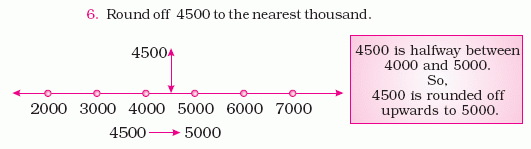Name: ___________________Date:___________________

 Method: Read out the numbers given. If the number in hundreds place is greater than 5, then the number is increased to the nearest thousands. If the number in hundreds place is a number less than 5, then it is reduced to the nearest thousands. If the number in hundreds place is a number equal to 5, then it is rounded up. Example: Round 5346 to the nearest thousand. Since the number in hundreds place is 3, the number is reduced to the nearest thousands. Answer: 5000 Example: Round 7883 to the nearest thousand. Since the number in hundreds place is 8, the number is increased to nearest thousands. Answer: 8000 Example: Round 4500 to the nearest thousand.Answer: 5000 Directions: Round the following numbers to the nearest thousand. Also write at least ten examples of your own.Name: ___________________Date:___________________